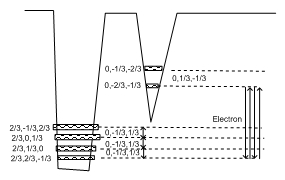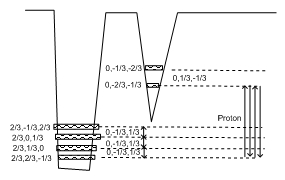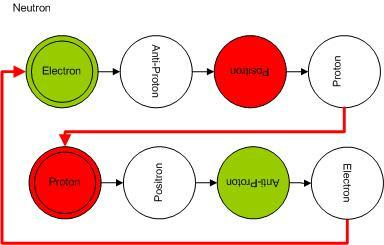# Hypergeometrical Universe

## Hyperluminal Neutrinos..:)

Hyperluminal Neutrinos

Today I will talk about a blockbuster research result from CERN. They discovered that Neutrinos can travel faster than the speed of light.  That has been in the News, hot from the Press...:) Earth shattering discovery.

Within the framework of the Hypergeometrical Universe theory,  a more fundamental theory, this higher than the speed of light amazing discovery is simply explained away with a simple phrase:

Hyperluminal Neutrinos  ==  Dispersion
That is Electron Neutrinos are just asymmetric mode dilatons

Einstein's made many great contributions to Science. The photoelectric effect; proving the existence of atoms by modeling Brownian motion. I have to say, even Restrict Relativity was a great achievement since it emphasized the need for an interpretation where observed (proper) time had to be expanded to explain the observed Universe. I consider General Relativity his least contribution due to the ad-hoc nature of his equations and the resulting confusion of equations with a pole.  They were the source of the Black Hole conjecture.

In the past, I went further and  explained how the passage of time is dependent upon which laws we choose to describe the Universe in The Image in the Mirror posting. Within the Hypergeometrical Universe, instead of Newton's Gravitational Law (the one with a horrendous pole at zero distance), I proposed that dilators (Hypergeometrical Universe "particles") dilate in phase with the surrounding dilaton field.  This is called the Quantum Lagrangian Principle and it is easily supported by considerations of metric deformation quantization (which lays a level deeper than our observed Quantum Mechanics).

By the way, that pole is the reason why we were misguided into considering the existence of a singularity or dressed singularity or Black Hole. The negation of the existence of a singularity naked or covered is different from saying that there isn't compact distributions of matter that irradiates little or no visible ligth.  This objection only refers to the spacetime representation with Mass Dependent Curvature.  This curvature is dependent upon what laws we use to describe motion. If you law has a pole, then the curvature or spacetime will have to have a singularity.  If you choose a law without a pole, there will be no need for a singularity.

The Quantum Fundamental Principle defines the position of dilators at each consecutive de Broglie step of expansion of the Hyperspherical Universe.  There is an accumulation point at 45 degrees with respect to the hyperspherical 3D universe. At that angle, matter is traveling at the speed of light and displacements at each de Broglie step approaches asymptotically zero, that is, no motion at time goes by on each and every other reference frame.  An dense assemble of dilators will create exact these conditions and light (which is generate by charge motion) will not be generated. In fact, the Black Hole should really be seemed at low frequency radiowaves from that line of reasoning.

All particles, with the exception of the Neutrino, are variations of the Fundamental Dilator and represent coherences with periods close to multiples of the Fundamental Dilator period.  This makes them strong interacting.  Since they contains the Fundamental Dilator as one of their sub-coherences, they contain local metric deformations that have non zero displacement volume per cycle  in nature.

The Neutrino, on the other hand, is a ellipsoid of revolution with only two non-null axes.  Since the axes have opposing values the total displacement volume per cycle is null and it also has the wrong frequency.

I haven't paid enough attention to the 4 dimensional ellipsoid of revolution physical model for the dilators, but I will try to make everything coherent there.  The physical representation of the dilators is not necessary for this theory to make sense.

Protons, Neutrons, etc have three non-null axes of revolution. Electron and Electron-Neutrino have only two. Electron has a non-zero displacement volume per cycle while neutrino has a zero displacement volume per cycle due to its opposing sign axes' lengths.

This will become clearer next.

Let's review the Neutron Model to make sense of what I just wrote.This was first present in the Review of the Hyperon Family Up to the Pion.

Review of Electron, Proton and Neutron Models

Let take a look at the Fundamental Dilator once again.

The Electron(Proton):The fundamental dilator is a 4D coherence between stationary states of deformation. The deformation of space is quantized, that is, can be represented as states in a 4D double potential well.

The respective coherence for Electron and Proton are shown below:and for a Proton:Notice that the Proton and Electron coherences differ only by which is the initial (in phase) state in the coherence. The states associated with the Electron (0,-2/3,-1/3) and (0,-1/3,-2/3) differ not by "energy" since they have the same 3D volume (the Hpergeometrical Universe equivalent to Energy is the 3D projection of the 4D Volume) but by time. It takes time to rotate the dilator around (at the speed of light) and that introduces an extra phase delay on the Tunnelling Process with respect to the Spinning Process. Remember that all particles in the Universe are described in terms of combinations of this two fundamental dilators and that they have Spin Half, that is, they spin at half the speed of tunneling...:) A nice touch, I might say...:)

The Neutron is the first dimmer or two chord sequence. Its Balls Diagram is shown below:Notice that I decided to use Red Balls for Positive and Green Balls for Negative moieties...:) Electrician Conventions... (never cut the Green Wire...)  :)

Notice that the diagram above introduce one transmutation chord:
• Electron-Proton Transmutation Chord

The dimmer character is derived from the impossibility to determine which dimensional note (Electron or Proton) is in phase with the Universe. Under these conditions the Neutron is represented by the two states (one starting with the electron and another starting with the proton) with half probability each one.

The Nature of Neutrinos

Below we show the Anti-Neutrino Coherence.
The anti-neutrino corresponds to the composite of the two transmutation chords found in the neutron coherence. That is the reason that if you split a neutrino, you will be able to create anti-mater out of Matter.
Someday I will explain that in a posting..:) Splitting Hairs and Neutrinos...:)

The 3D Volume Displacement equation is given by:
Neutron(1/3,1/3,-2/3) = Proton(2/3,2/3,-1/3) + Electron(0,-1/3,-2/3) + Anti-Neutrino(-1/3,0,1/3)

That means, unlike the electron which is also has only two axes but corresponds to a net decrease in local metric displacement volume (decrease in one phase but a net increase in the alternative phase when protons become a net decrease), the neutrino is always a net zero local metric displacement volume change.  In the example above, the anti-neutrino corresponds to a shrinkage along the X dimension and an stretch on the Z dimension.  This local metric modulation changes the moment of inertia of the sub-coherence and allow for the electron-proton transmutation.  The local metric modulation changes the moment of inertia and in doing so changes the timing between tunneling and spinning within the four-dimensional spatial manifold.

The anti-neutrino corresponds to the electron-proton + proton-electron transmutation.  I struggled to figure out if that sub-coherence was supposed to be in the lower states or in the upper states.

I had to decide if the transmutations corresponds to this sequence
(0,-2/3,-1/3) > (2/3,2/3,-1/3)>(2/3,-1/3,2/3)>(2/3,2/3,-1/3)
or
(0,-2/3,-1/3)>(0,-1/3,-2/3)>(0,-2/3,-1/3)>(2/3,2/3,-1/3)
that is, I had to decide if the delay was cause by a 3D rotation (rotation within the 3D hypersurface) while the coherence was in the lower or in the upper state (proton or electron states).

After looking at the assignments for all the hyperons and analyzing all hyperon decay equations, I decided that the anti-neutrino (electron-neutrino) would correspond to a 3D rotation (rotation within the 3D Hypersurface) while the neutron coherence was in the proton state, to my surprise.:)  I was hoping for a neater result, that is, that the anti-neutrino would correspond to a rotation while the dilator coherence is in the electron state..:) but such is life... full of disappointments..:)

Now we know that the neutrino corresponds to a coherence involving the following diagram
it becomes easier to understand the relationship between this diagram and the (-1/3,0,1/3) representation.

If I shrink everything along X and and equally stretch everything along Z, that would correspond to a rotation around the Y axis, or a rotation in the 3D space.

Now let's compare the Neutrino with light.

In my theory light is a modulation of the source position of the dilaton field. The dilaton field is continuously irradiated without loss of energy.  In the same way an electromagnetic field would be irradiated without loss if there weren't any absorbers around.  For an electromagnetic coherence to decay and energy be absorbed or irradiated, a dephasing event has to occur. Like in the case of a Boy in the Swing, if one doesn't push the swing in an out-of-phase fashion, energy is never lost nor absorbed (disregarding friction). The propagation of the dilaton field occurs in a pristine 4D spatial manifold without anything to absorb that dilaton field.

Both have zero net metric displacement per cycle. They differ in the nature of their modes.  Like electromagnetic modes, electromagnetic waves are just modulations of a modulation (the dilaton field).  The dilaton field can be thought as a TEM00 or totally symmetric mode. Of course, the Electron Neutrino can be thought as the first asymmetric mode.  The dilaton mode is not transversal, it is volumetric. The Neutrino mode is asymmetric and transversal.

In the case of deformation of the local metric, one would expect DISPERSION, that is, different modes with different coherences and frequencies would travel at different speeds, thus the difference in speed between Neutrinos and Photons.

Please feel free to ask me questions.  They will help me to clarify the points in this posting.

QED.

Cheers,

MP

PS_ I refreshed the version of my theory, that is, the PDF in this page corresponds to the paper I presented at the 7th Vigier Symposium in London last year.
Currently unrated

or